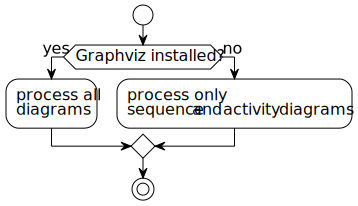# Speedcoding

Tips on how to solve an interview problem within 20 minutes.

## Strategy Diagram## Writing Code

### Shortcuts

• Ctrl + `/` comments out code; pressing again removes comments
• Ctrl + `[` increases indentation, while `]` decreases indentation

### Default Includes

``````#include <iostream>

#include <algorithm> // std::sort, std::find, std::copy, std::min_element, std::max_element
#include <functional> // std::greater
#include <span>
#include <ranges>

#include <limits>
#include <numbers>

#include <array>
#include <map>
#include <unordered_map>
#include <set>
#include <unordered_set>
#include <vector>
#include <tuple>
#include <utility>

#include <string>
#include <string_view>

#include <mutex>
#include <memory>

#include <filesystem>

#include <any>
#include <variant>
``````

### `std` Namespace

Avoid typing `std::` over and over again.

``````using namespace std;
``````

### Builtins

• `__builtin_clz` returns the number of leading zeroes.

• `__builtin_ctzl` for `long`.
• `__builtin_ctzll` for `long long`.
• `__builtin_ctz` returns the number of trailing zeroes.

• `__builtin_ctzl` for `long`.
• `__builtin_ctzll` for `long long`.
• `__builtin_parity` returns 1 if the number is odd, 0 otherwise.

• `__builtin_parityl` for `long`.
• `__builtin_parityll` for `long long`.
• `__builtin_popcount` returns the number of ones.

• `__builtin_popcountl` for `long`.
• `__builtin_popcountll` for `long long`.

### `auto` Variable Type

``````auto i = 0;
``````

`auto` also works in function return types, as well as lambda parameters as of C++14.

### Range-based `for` Loop

``````vector<int> v = {1, 2, 3};

for (const auto& el : v) {
cout << el;
}
``````

### Ranges

``````sort(v.begin(), v.end());
// vs.
ranges::sort(v);
``````

### Views

``````vector<int> v{1, 2, 3, 4, 5};

// You can pipe `|` multiple filters.
auto greaterthan2view = v | views::filter([](int x){return x > 2;});
``````

### Beautify Code

Use single quotes to separate digits.

``````iny x = 100'000'000;
``````

### Avoiding Overflow

If you're looking for a number `a` divisible by `b` then it's recommended to work in modulo `b` operations to avoid overflow. Please see the binary prefix divisible by 5 problem on LeetCode.

### Counting Digits

``````floor(log10(n) + 1) // represents the number of digits in base 10 of `n`.
``````

## Testing Code

### Asserts

``````auto a = 10;

static_assert(a == 10, "`a` should be 10 at compile-time.");
``````

## Debugging Code

Print the items from a vector:

``````vector<int> list;

for_each(list.begin(), list.end(), [](auto& item){ cout << item << endl; });
``````

Print the items from an unordered map:

``````unordered_map<int, int> map;

for_each(map.begin(), map.end(), [](auto& item){ cout << item.first << " " << item.second << endl; });
``````

### Disabling Standard Out

Keep the `cout` statements in code and easily toggle them on or off.

``````cout.setstate(ios_base::failbit);

cout << "Hello World" << endl; // will print nothing
``````

## Improving Code

### GCC Optimizations

``````#pragma GCC optimize("Ofast")
#pragma GCC optimization("unroll-loops")
#pragma GCC optimization("max-inline-insns-recursive-auto")
#pragma GCC optimization("fast-math")

#pragma GCC target("avx,avx2,fma")
``````

### Attributes

Use `[[likely]]` and `[[unlikely]]` attributes to inform the compiler which statements are likely, and respectively unlikely to be executed more often.

``````if (a % 2 == 0) [[likely]] {
cout << "`a` is even." << endl;
} else [[unlikely]] {
cout << "`a` is odd." << endl;
}
``````

### Input Output (IO)

Makes use of lambda functions to not have C input sync with C++.

``````static const int _=[](){ios::sync_with_stdio(false);cin.tie(nullptr);cout.tie(nullptr);return 0;}();
``````

## Communication

### ASCII Art

Using `^` to point to where the cursor is located can help interviewers understand what you're explaining.

``````auto s = "abcdef";
//          ^
``````

## Tricks

Moving all zeroes at the end of array.

``````stable_partition(nums.begin(), nums.end(), [](int n){ return n != 0; });
``````